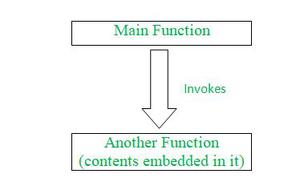# How to create a function that invokes function with partials prepended arguments in JavaScript ?

• Difficulty Level : Easy
• Last Updated : 27 Jul, 2021

In this article, we will see how to create a function that invokes functions with partials prepended to the arguments it receives in JavaScript. Before understanding the problem statement and approaching the solution for the same, let’s, first of all, know what a function (also called a method) is and how a function gets executed.

A function (or a method) is a block of code that performs a particular task for which the function is made. A function can be called any number of times inside the program to perform that particular task. Dividing a large-sized code into a small block of code not only increases the readability of the code but also helps the developer to find the bug in the particular section of the code. This makes the program net & clean as well as makes it more authentic. Basically, for executing a particular task for more than one time, we can write our logic part in a separate function (method) and can use that function whenever we need it in a program.

There are many ways by which we can create a simple function (method), like by using the function keyword or creating arrow functions, the syntax would be a little different in both cases, but eventually, the result will remain the same. The following is the syntax by which we can declare our function (method) and execute it further-

## Javascript

 `function` `displayName (name) {``    ``return` `name;``}``console.log(displayName(``"GeeksforGeeks"``));`

The output of the above code would be as follows-

`GeeksforGeeks`

We may declare our method or function in the form of arrow functions (ES6 version format) by using the following syntax-

## Javascript

 `let displayName = (name) =>{``    ``return` `name;``}``console.log(displayName(``"GeeksforGeeks"``));`

The output of the above code would be as follows-

`GeeksforGeeks`

Now let’s understand the problem statement, for which we need to find out the particulars too. The following pictorial representation describes the problem statement-We need to create a function that is responsible for invoking another function which consists of several partials (contents) prepended to the arguments in it. A user thus uses the main function to display the result which will indirectly use another function containing information. Now that we have understood the problem statement, then we will move further. We will now see different approaches to solve this particular problem.

The following are the approaches through which we could solve our problem with ease-

Approach 1:

• This approach is the first basic as well as the native approach.
• Here, we will create several functions which we require to execute our problem statement and get our results.
• We will create a function using the “function” keyword which we use in JavaScript for any function creation.
• Eventually, we will return the result of our nested functions so that it gets stored into the main function.
• First, we would create a function which is the main function named partial which would contain another function inside its list of arguments and, further, we would use the rest operator “…” along with a variable which would indicate that along with the function you may pass on an indefinite list of arguments in a partial function.
• Afterwards, inside that partial function, we would be returning to an anonymous function which would again be containing a rest operator along with the “args” variable, which indicates that indefinite arguments could be accepted with ease.
• Further, we would be returning to the function named “fn” which we had passed inside the main function named partial, which would eventually return the rest operator with two variables that would eventually accept an indefinite list of arguments.
• Afterwards, we would use the above-declared function in such a way by creating another function for our ease which would accept two parameters names greeting and name which would be passed by the user itself.
• Then we would store our result in a variable called greetMessage wherein we would pass our main method along with a static greeting message and furthermore, the name would be passed on while printing the result on the console.

## Javascript

 `function` `partial(fn, ...partials) {``  ``return` `function` `(...args) {``    ``return` `fn(...partials, ...args);``  ``};``}`` ` `function` `greet(greeting, name) {``  ``return` `greeting + ``" "` `+ name;``}`` ` `let greetMessage = partial(greet, ``"Hello"``);`` ` `console.log(greetMessage(``"GeeksforGeeks"``));`

The output of the above code snippet would be as follows-

`Hello GeeksforGeeks`

Approach 2:

• This second approach is quite a better approach as well as the simplest one.
• In this, we will be using arrow functions (ES6 feature of JavaScript).
• We will create one main function and inside that main function, we will just pass in several other arrow functions so as to execute the result in the middle nested functions which get stored into the main function.
• We will create arrow functions one after another. In the end, we would be creating a variable that will store our result and thus, with the help of that particular variable would get our result on the console.
• The steps which are described in the first approach would remain exactly the same and the only thing which will be changed in this approach is just the use of arrow functions here, which would make our code look a little shorter, more readable and understandable.

## Javascript

 `let partialFunction = (fn, ...partials) => (``    ``...args) => fn(...partials, ...args);`` ` `let greet = (greeting, name) => greeting + ``" "` `+ name;`` ` `let greetMessage = partialFunction(greet, ``"Hello"``);`` ` `console.log(greetMessage(``"GeeksforGeeks"``));`

The output of the above code snippet would be as follows-

`Hello GeeksforGeeks`

Hey geek! The constant emerging technologies in the world of web development always keeps the excitement for this subject through the roof. But before you tackle the big projects, we suggest you start by learning the basics. Kickstart your web development journey by learning JS concepts with our JavaScript Course. Now at it’s lowest price ever!

My Personal Notes arrow_drop_up